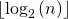# Practice Questions for Recursion | Set 2

Explain the functionality of the following functions.

Question 1

## C++

 `/* Assume that n is greater than or equal to 1 */` `int` `fun1(``int` `n)` `{` `    ``if` `(n == 1)` `        ``return` `0;` `    ``else` `        ``return` `1 + fun1(n / 2);` `}`

## Java

 `/* Assume that n is greater than or equal to 1 */` `static` `int` `fun1(``int` `n)` `{` `    ``if` `(n == ``1``)` `        ``return` `0``;` `    ``else` `        ``return` `1` `+ fun1(n / ``2``);` `}`

## Python3

 `# Assume that n is greater than or equal to 1 */` `def` `fun1(n):` `    ``if``(n ``=``=` `1``):` `        ``return` `0` `    ``else``:` `        ``return` `1` `+` `fun1(n``/``/``2``)`

## C#

 `/* Assume that n is greater than or equal to 1 */` `static` `int` `fun1(``int` `n)` `{` `    ``if` `(n == 1)` `        ``return` `0;` `    ``else` `        ``return` `1 + fun1(n / 2);` `}`

## Javascript

 ``

Answer: The function calculates and returnsFor example, if n is between 8 and 15 then fun1() returns 3. If n is between 16 to 31 then fun1() returns 4.

Question 2

## C++

 `/* Assume that n is greater than or equal to 0 */` `void` `fun2(``int` `n)` `{` `if``(n == 0)` `    ``return``;`   `fun2(n/2);` `cout << n%2 << endl;` `}`

## C

 `/* Assume that n is greater than or equal to 0 */` `void` `fun2(``int` `n)` `{` `  ``if``(n == 0)` `    ``return``;`   `  ``fun2(n/2);` `  ``printf``(``"%d"``, n%2);` `}  `

## Java

 `/* Assume that n is greater than or equal to 1 */` `static` `void` `fun2(``int` `n)` `{` `if``(n == ``0``)` `    ``return``;` ` `  `fun2(n/``2``);` `System.out.println(n%``2``);` `} `

## Python3

 `# Assume that n is greater than or equal to 0 */` `def` `fun2(n):` `    ``if``(n ``=``=` `0``):` `        ``return` `    `  `    ``fun2(n ``/``/` `2``)` `    ``print``(n ``%` `2``, end``=``"")`

## C#

 `void` `fun2(``int` `n) ` `{ ` `if``(n == 0) ` `    ``return``; ` `  `  `fun2(n/2); ` `Console.Write(n%2); ` `} `

## Javascript

 ``

Auxiliary Space: O(log2N), due to recursion call stack

Time Complexity: O(log N)

Answer: The function fun2() prints the binary equivalent of n. For example, if n is 21 then fun2() prints 10101.

Note: Above functions are just for practicing recursion, they are not the ideal implementation of the functionality they provide.